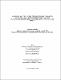## Numerical solutions of the inertial modes of the earth's fluid core : from the outstanding problem of the incompressible fluid shell to more realistic up to date core models2015
Naseri, Hossein
##### Publisher
Lethbridge, Alta. : University of Lethbridge, Dept. of Physics and Astronomy
##### Abstract
In this work we study the inertial modes of a rotating spheroidal fluid shell proportional to the Earth's fluid core. We start with the long standing problem of the modes of an incompressible and inviscid spherical fluid shell. Traditionally, a second order equation describing the pressure field of the flow, subject to the impermeability boundary condition, is solved for the eigenfrequencies and eigenfunctions of the flow. These equations are scalar hyperbolic boundary value second-order Partial Differential Equations (PDEs) which are ill-posed problems in the sense that the existence of the analytical solutions depends on the geometry of the container. The problem admits analytical solutions in a sphere but not in a spherical shell. We use the Galekin method to solve the momentum and the continuity equation together and compute the frequencies, pressure and displacement eigenfunctions for some of the low order, wavenumbers $m=0$ and $m=1$, inertial modes of this model. To show that our approach is correct we compute the inertial modes of a homogeneous, incompressible and inviscid fluid sphere for which analytical solutions for the inertial modes exist. We also compute the inertial modes of a more realistic uniformly rotating, compressible, self gravitation and inviscid fluid core model. Finally, we extend the governing equations to include first order terms in the ellipticity. In order to minimize effects of the derivatives of the material properties which are poorly determined in the existing Earth models, a Clairaut coordinate system is used to map the elliptical equipotential surfaces into the spherical ones. Also, the divergence theorem is used to implement the boundary equations.
##### Keywords
earth's core , rotating fluids , inertial modes , hydrostatic equilibrium , Galerkin method , compressibility , elastic boundary conditions , ellipsoidal core , Clairaut coordinates